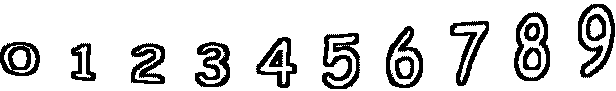7 + 4 =        4 + 5 =6 + 6 =        9 + 8 =        3 + 6 =        2 + 0 = 1 + 10 =        5 + 5 =        3 + 6 =        9 + 4 =